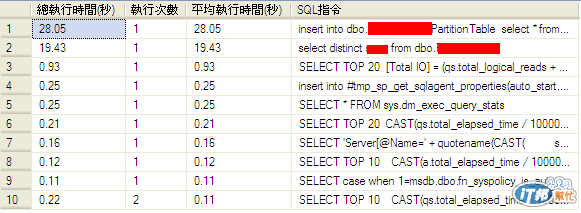#DAY 21
8

## Day21_使用dmv找出執行最久的查詢

``````SELECT TOP 10
[總執行時間(秒)]		=CAST(a.total_elapsed_time / 1000000.0 AS DECIMAL(16, 2))
, [執行次數]				=a.execution_count
, [平均執行時間(秒)]	=CAST(a.total_elapsed_time / 1000000.0 / a.execution_count AS DECIMAL(16, 2))
, [SQL指令]				=SUBSTRING (b.text,(a.statement_start_offset/2) + 1,500)
FROM sys.dm_exec_query_stats a CROSS APPLY sys.dm_exec_sql_text(a.sql_handle) as b
WHERE a.total_elapsed_time > 0 AND B.[text] NOT LIKE '%SCHEMA_NAME%'--去除一些系統的SQL指令
ORDER BY [平均執行時間(秒)] DESC
``````